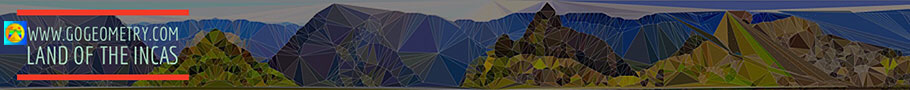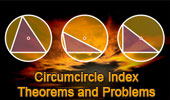# Plane Geometry: Circumcircle, Circumradius of a triangle, Theorems and Problems 8

 Circumcircle of a triangle - Table of Content 8Proposed Problem 82. Circumcircle, Circumcenter. Area of the Contact Triangle, inradius, circumradius. Proposed Problem 81. Circumcircle, Circumcenter. Area of a triangle, side, inradius, circumradius. Proposed Problem 67: Circumcenter Triangle, Circumcircle, Angles, Cyclic Quadrilateral. Miquel's Pentagram with Dynamic Geometry. Circumcircles, Circumcenters. You can alter the pentagram dynamically in order to test and prove (or disproved) conjectures and gain mathematical insight that is less readily available with static drawings by hand. Miquel's Pentagram Theorem Interactive proof with animation and key theorems. Circumcircles, Circumcenters. Adams' Circle Theorem. Circumcircle, Circumcenter of the contact triangle Lemoine Theorem. Circumcircle, Tangent, Collinear points. Sawayama -Thebault's theorem. Circumcircle, Incircle, Collinear points. The Bevan Point The circumcenter of the excentral triangle. Illustration with animation. Circumcircle. The Bevan Point: Puzzle The circumcenter of the excentral triangle. Circumcircle. Go to page: Previous | 1 | 2 | 3 | 4 | 5 | 6 | 7 | 8 | Next

 Home | Search| Geometry | Triangles | Centers | Post a comment | Email | by Antonio Gutierrez